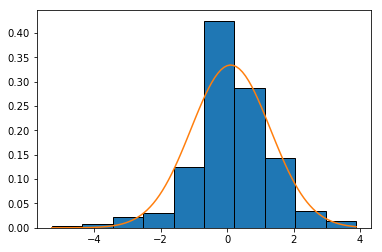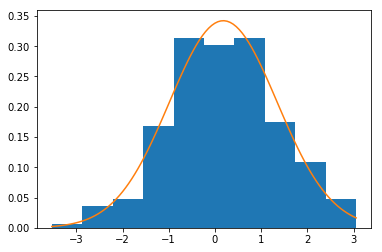# Data Analysis with Python¶

In :
# 1. import relevant libraries
import numpy as np
import pandas as pd
import matplotlib.pyplot as plt
import pandas_datareader as pdr
%matplotlib inline

In :
# 2.  Download stock price data, store in dataframe
amzn = pdr.get_data_google('AMZN', start='2016-08-03')

In :
# 3. Take a look at the data
amzn.head()

Out:
Open High Low Close Volume
Date
2016-08-03 757.06 758.89 752.25 754.64 3581525
2016-08-04 753.70 765.00 750.35 760.77 3178208
2016-08-05 764.81 768.47 763.09 765.98 2704391
2016-08-08 766.81 767.00 761.02 766.56 1986272
2016-08-09 767.39 772.60 766.90 768.31 1876091
In :
# 4. Get just the data we are interested in
amzn_close = amzn['Close']

In :
# 5. Verify data
type(amzn_close.head())

Out:
pandas.core.series.Series
In :
# 6. Convert close-to-close dollar change into instantaneous rate of return, verify transformation
amzn_percent_change = np.log(amzn_close / amzn_close.shift(1)) * 100
amzn_percent_change.head()

Out:
Date
2016-08-03         NaN
2016-08-04    0.809026
2016-08-05    0.682498
2016-08-08    0.075691
2016-08-09    0.228032
Name: Close, dtype: float64
In :
# 7. Pandas describe function gives descriptive statistics, default assumes you are dealing with a sample
# Pandas also deals with missing values by ommitting them
amzn_percent_change.describe()

Out:
count    252.000000
mean       0.106487
std        1.194826
min       -5.273753
25%       -0.484134
50%        0.117312
75%        0.811309
max        3.881057
Name: Close, dtype: float64
In :
# 8. An alternative more comprehensive table of descriptives can be generated using scipy stats, assumes a sample
from scipy import stats
stats.describe(amzn_percent_change[1:])

Out:
DescribeResult(nobs=252, minmax=(-5.2737531271995062, 3.8810569610031007), mean=0.10648736829713538, variance=1.4276100656074371, skewness=-0.5610474712532317, kurtosis=2.3600263221846784)
In :
# 9. for comparision generate random numbers that follow normal distribution
x = np.random.normal(.1064,1.1948, 252)
stats.describe(x)

Out:
DescribeResult(nobs=252, minmax=(-3.530203911382729, 3.0549917950041023), mean=0.18017895760082278, variance=1.364946888190105, skewness=-0.05145305633122407, kurtosis=-0.1534361303525733)
In :
# 10. use scipy stats to test the kurtosis, the null hypothesis is that the sample is drawn from a population
# where the underlying kurtosis is that of a normally distributed variable
print("x: ", stats.kurtosistest(x))
print("amzn: ", stats.kurtosistest(amzn_percent_change[1:]) )

x:  KurtosistestResult(statistic=-0.33790480941260126, pvalue=0.73543492313774006)
amzn:  KurtosistestResult(statistic=4.2481322914200996, pvalue=2.1556009784047011e-05)

In :
# 11. plot histogram of price changes with normal curve overlay
import matplotlib.mlab as mlab

plt.hist(amzn_percent_change[1:], edgecolor='black', normed=True)

# overlay normal curve
mean = float(np.mean(amzn_percent_change))
sd = float(np.std(amzn_percent_change, ddof=1))
min_chng = float(np.min(amzn_percent_change))
max_chng = float(np.max(amzn_percent_change))
a = np.linspace(min_chng, max_chng,100)
plt.plot(a,mlab.normpdf(a,mean,sd))

Out:
[<matplotlib.lines.Line2D at 0x115a03e80>]In :
# 12. compare to sample drawn from a normally distributed population with same attributes as amzn price change
plt.hist(x, normed=True)

mean = float(np.mean(x))
sd = float(np.std(x))
b = np.linspace(np.min(x), np.max(x), 100)
plt.plot(b,mlab.normpdf(b, mean, sd))
plt.show()In [ ]: